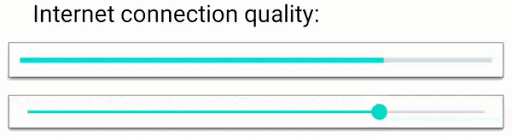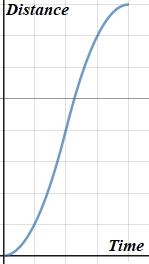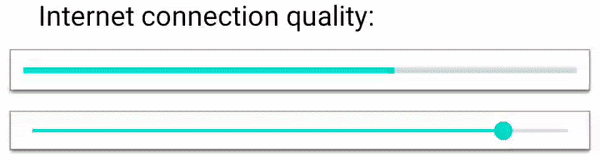# Animating a stream of values in Android

Let’s imagine the situation: you have a stream of numeric values and you want to illustrate it in a View. Standard Android animators are intended for transition from the initial to target state with fixed duration and cannot work with a stream of values. In this article we will explain how to create custom animators for live data changes and interpolate them.

## Problem description

Consider that we have a service that provides a value that changes several times per second (for example, internet connection quality, in percent), and we want to display it in a View (e.g. ProgressBar). Let’s first write code that does it without any animations:

TrackInternetConnectionQualityService.addInternetConnectionQualityListener { quality ->
progressBar.progress = quality
}


The result will look like this:Obviously, this animation needs smoothness. If we use one of the standard Android animators, the displayed value will be interpolated from the very beginning every time a new number is emitted. Resetting any default Android animator on every change will cause unwanted “jumps” since the previously displayed value will be lost. So this method is only useful if the stream emits infrequently and we use a linear interpolator.

## Solution

How to animate a View without using default animators? By creating your own! Firstly, we need to create a callback for frame updates. For this purpose, Android provides Choreographer#postFrameCallback and View#postOnAnimation methods. Using the latter, let’s create a method that is called on each frame and reduces the difference with the target value by a few percent, say by 8:

var targetValue = 0f
var currentValue = 0f
val fractionPerFrame = 0.08f

private fun postNextFrame() {
progressBar.postOnAnimation {
onNewFrame()
}
}

private fun onNewFrame() {
postNextFrame()
val diff = targetValue - currentValue
currentValue += diff * fractionPerFrame
progressBar.progress = currentValue.roundToInt()
}


And value callback now looks like this:

TrackInternetConnectionQualityService.addInternetConnectionQualityListener { quality ->
targetValue = quality
}


As a result, we get smooth value changing:Here the bottom bar illustrates the target value changes without animation.

However, this method does not use frame time for calculation, so the animation speed will depend on the frame rate of the device. Let us improve it by creating a ValueStreamAnimator class using Choreographer.

class ValueStreamAnimator<T>(
initialValue: T,
private val propertySetter: (T) -> Unit,
private val interpolator: (currentValue: T, targetValue: T, timeMills: Long) -> T
) {
private val choreographer = Choreographer.getInstance()
var targetValue = initialValue
private var currentValue = initialValue
private var isStarted = false

private fun postNextFrame() {
choreographer.postFrameCallback { nanoTimeOnPreviousFrame ->
onNextFrame(((System.nanoTime() - nanoTimeOnPreviousFrame) * 1e-6).roundToLong())
}
}

fun start() {
if (!isStarted) {
isStarted = true
postNextFrame()
}
}

fun stop() {
isStarted = false
}

private fun setValue(value: T) {
propertySetter(value)
currentValue = value
}

private fun onNextFrame(frameTimeMills: Long) {
if (!isStarted) {
return
}
postNextFrame()
setValue(interpolator(currentValue, targetValue, frameTimeMills))
}
}


It is only a base for such animators and could be modified according to your requirements. Here propertySetter parameter is responsible for updating the value (in our case setting progress to ProgressBar).

In this implementation we can’t use fixed start/end values for interpolation, because they are absent for infinite streams. Thus, we have to work with live parameters and use current value, target value and frame time instead to calculate the next displayed value. interpolator parameter defines this mapping.

This implementation is based on an endless recursion, which has to be manually stopped by calling stop() method. To avoid bothering with that and to make animation stop automatically after target view destruction, we can use a WeakReference of it (like in ObjectAnimator).

class ValueStreamAnimator<T, V: View>(
initialValue: T,
targetView: V,
private val propertySetter: (T, V) -> Unit,
private val interpolator: (currentValue: T, targetValue: T, timeMills: Long) -> T
) {
private val viewReference = WeakReference<V>(targetView)
...
private fun setValue(value: T) {
val view = viewReference.get()
if (view == null) {
stop()
return
}
propertySetter(value, view)
currentValue = value
}
...
}


## Interpolators

The bottleneck of this solution is complex interpolation, because we have to implement it ourselves. To create a common Linear interpolator, we should introduce the speed of value changing. For a property of Float type it will look this way:

val speed = 1.5f
val interpolator = { currentValue: Float, targetValue: Float, timeMills: Long ->
val difference = targetValue - currentValue
val maxDifferencePerTime = timeMills * speed
currentValue + difference.coerceIn(-maxDifferencePerTime, +maxDifferencePerTime)
}To create a Logarithmic interpolator, where each frame the difference with the target value is reduced by a fixed fraction as in the very first example, we should use pow function:

val fractionPerMillisecond = 0.07f
val interpolator = { currentValue: Float, targetValue: Float, timeMills: Long ->
val difference = targetValue - currentValue
targetValue - difference * (1 - fractionPerMillisecond).pow(timeMills.toInt())
}


In this example we pass 7% of the remaining distance to target each millisecond.To avoid instant speed changes we can use the most complex interpolator – AccelerateDecelerate interpolator. To achieve this we should on each frame increment or decrement speed by a fixed value – acceleration. And when we reach the target value, speed should become zero. The graph below illustrates distance changes.Let $V_{cur}$ be the current speed and $a$ be the absolute acceleration ($+a$ when speed is increasing, $-a$ when decreasing). Then the total distance $d$ (difference between current and target values) can be calculated as

using the fact that the speed first increases, then decreases and becomes zero at the end. Here $T_{inc}$ and $T_{dec}$ are the durations of speed increasing and decreasing respectively, which we would like to find.

On the other hand, $aT_{dec} = V_{cur} + aT_{inc} \Rightarrow T_{dec}=\frac{1}{a}V_{cur} + T_{inc}$, where acceleration should have the same sign as difference. Substituting the expression for $T_{dec}$ into the formula for the difference we have:

We got a quadratic equation for $T_{inc}$, which can be solved using the discriminant:

We need to find a positive solution, for this we should use the discriminant with the same sign as acceleration. Otherwise the speed after increasing will be negative.

If this equation has no solutions, then the current speed is too big, and we don’t have to increase it.

val absoluteAcceleration = 0.1f
var currentSpeed = 0f
val interpolator = { currentValue: Float, targetValue: Float, timeMills: Long ->
val difference = targetValue - currentValue
val acceleration = difference.sign * absoluteAcceleration

var deltaTime = timeMills.toFloat()
var newValue = currentValue

val increaseTime = min( // increaseTime can not be greater than time we have
(-currentSpeed + difference.sign * sqrt(currentSpeed.pow(2) / 2 + acceleration * difference)) / acceleration,
deltaTime
)
if (increaseTime > 0) {
deltaTime -= increaseTime
newValue += increaseTime * (currentSpeed + acceleration * increaseTime / 2)
currentSpeed += increaseTime * acceleration
}
val decreaseTime = min(abs(currentSpeed / acceleration), deltaTime)
if (decreaseTime > 0) {
deltaTime -= decreaseTime
newValue += decreaseTime * (currentSpeed - acceleration * decreaseTime / 2)
currentSpeed -= acceleration * decreaseTime
}

newValue // return value
}


As a result, rapid target value changes do not cause instant speed changes:## Conclusion

In this article we explained how to create a custom animator for a stream of numeric values and showed how to implement the most common interpolators for it. These ideas can be used for any platform, not only Android. You can also smooth movements in any-dimensional field using animators for each coordinate separately. Note that in these examples we used randomly generated values and in real cases it’s better to try all interpolators and pick the most appropriate one.